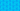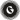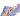PricingResources

# What are semi-variable costs?Written by

Semi variable costs refer to costs incurred by a company, which is a combination of fixed and variable costs. Also known as mixed cost or semi-fixed cost, this type of cost is common across several industries and sectors. Keep reading to know more about semi-variable cost and its examples.

## Semi-Variable Cost Explained

Generally, a business is said to incur two types of cost – fixed cost and variable cost. The fixed cost refers to a cost that doesn’t change regardless of the production output. In contrast, a variable cost is one that depends solely on the level of output. A semi-variable cost therefore combines the features of a fixed cost and a variable cost.

Typically, production of goods or service-offering up to a specific limit is governed by a fixed cost. Regardless of the production output, as long as it falls within the specified limit, it continues to incur a fixed cost.

But when the set level is exceeded, the cost that governs the production is then referred to as a variable cost. So, under a semi-variable cost, a variable cost component arises where a specific limit has been exceeded.

Generally, the semi-variable cost is relevant for the projection of financial performance across the different production lines.  For instance, where the fixed cost in a semi-variable cost is lower, this suggests that the business has a lower break-even point and can easily achieve break-even.

It is worth remembering that a semi-variable cost is an accounting principle. But unlike fixed cost and variable cost, companies don't provide independent details of their semi-variable cost in their financial statements. Instead, they are classified into other related categories. This is because identifying a semi-variable cost is usually relevant for internal purposes only, unlike fixed and variable costs that serve external purposes as well.

## Semi-variable Cost Examples

A good example of semi-variable cost can be found in the cost of operating a vehicle. Generally, the cost of the vehicle is fixed regardless of its level of usage. This comprises depreciation, insurance and the driver's monthly salary.

In contrast, other costs associated with the vehicle’s productivity level in a period, such as fueling the vehicle, represent the variable cost. All these costs then give rise to the semi-variable cost of the vehicle.

Another semi-variable cost example is where the company has an industrial machine for sewing. In this instance, the cost of insurance, licensing, and depreciation of the industrial sewing machine is fixed and does not change regardless of the output.

On the other hand, the cost of fueling the machine and the cost of the thread used are examples of variable costs. This is the same with other costs of maintenance incidental to its continuous use. A combination of these costs using the semi-variable cost formula will produce the semi-variable cost.

## How to Calculate Semi-Variable Cost

The semi-variable cost formula looks like this:

So, for example, where the vehicle’s fixed cost totals £200, 00000, and the cost of fueling the vehicle is £200 per hour, and the vehicle spends 350 hours on the road in a month, the semi-variable cost is as follows:

Semi-variable cost (£270,000) = fixed cost (£200,000) + unit variable cost (£200) unit (350)

Here: Unit variable cost (£200) x unit (350) = £70,000

## We Can Help

GoCardless helps you automate payment collection, cutting down on the amount of admin your team needs to deal with when chasing invoices. Find out how GoCardless can help you with ad hoc payments or recurring payments.Contact sales

Sales

Support